Скачать презентацию 21 Theory of Consumer Choice PRINCIPLES OF FOURTH

766069cd64b3dd7334de64b5377b90f9.ppt

• Количество слайдов: 49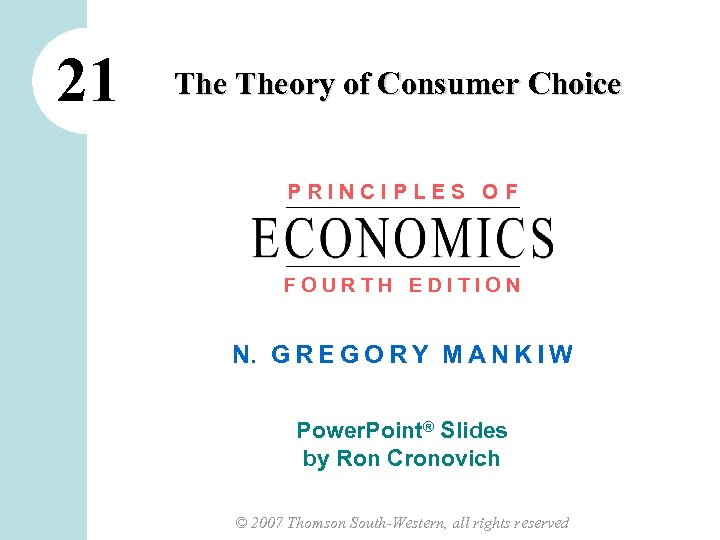21 Theory of Consumer Choice PRINCIPLES OF FOURTH EDITION N. G R E G O R Y M A N K I W Power. Point® Slides by Ron Cronovich © 2007 Thomson South-Western, all rights reserved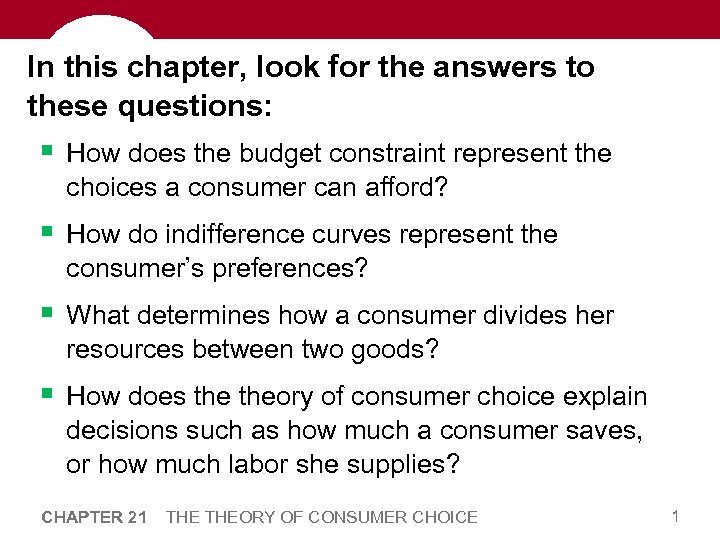In this chapter, look for the answers to these questions: § How does the budget constraint represent the choices a consumer can afford? § How do indifference curves represent the consumer’s preferences? § What determines how a consumer divides her resources between two goods? § How does theory of consumer choice explain decisions such as how much a consumer saves, or how much labor she supplies? CHAPTER 21 THEORY OF CONSUMER CHOICE 1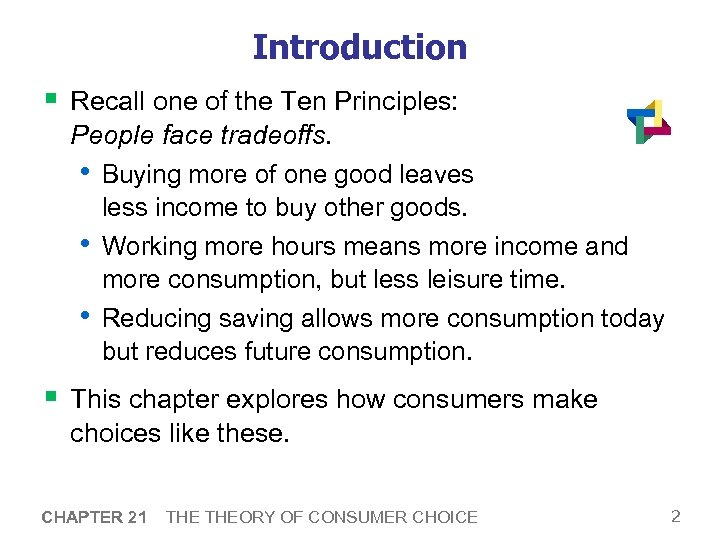Introduction § Recall one of the Ten Principles: People face tradeoffs. • Buying more of one good leaves less income to buy other goods. • Working more hours means more income and more consumption, but less leisure time. • Reducing saving allows more consumption today but reduces future consumption. § This chapter explores how consumers make choices like these. CHAPTER 21 THEORY OF CONSUMER CHOICE 2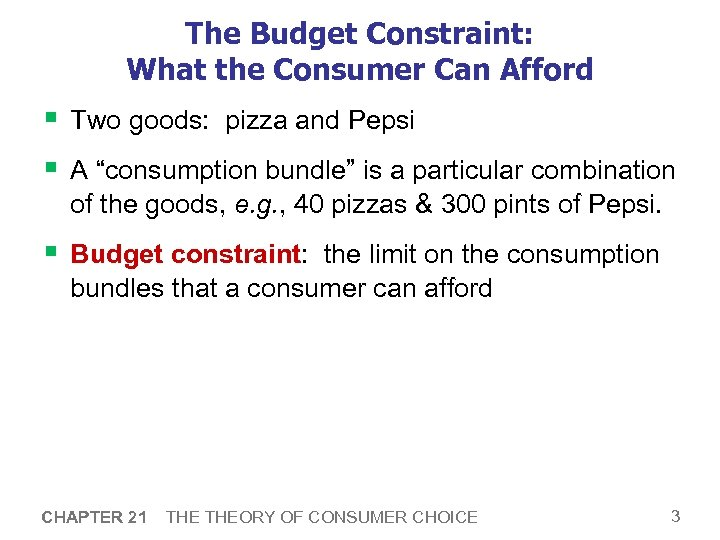The Budget Constraint: What the Consumer Can Afford § Two goods: pizza and Pepsi § A “consumption bundle” is a particular combination of the goods, e. g. , 40 pizzas & 300 pints of Pepsi. § Budget constraint: the limit on the consumption bundles that a consumer can afford CHAPTER 21 THEORY OF CONSUMER CHOICE 3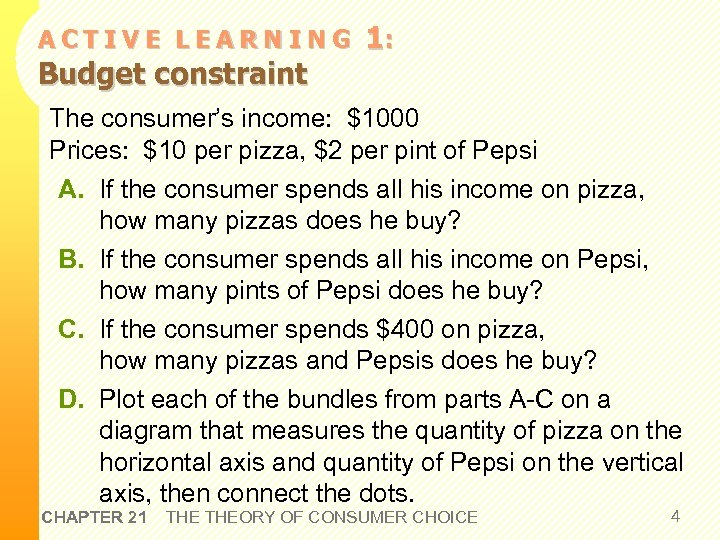ACTIVE LEARNING Budget constraint 1: The consumer’s income: \$1000 Prices: \$10 per pizza, \$2 per pint of Pepsi A. If the consumer spends all his income on pizza, how many pizzas does he buy? B. If the consumer spends all his income on Pepsi, how many pints of Pepsi does he buy? C. If the consumer spends \$400 on pizza, how many pizzas and Pepsis does he buy? D. Plot each of the bundles from parts A-C on a diagram that measures the quantity of pizza on the horizontal axis and quantity of Pepsi on the vertical axis, then connect the dots. CHAPTER 21 THEORY OF CONSUMER CHOICE 4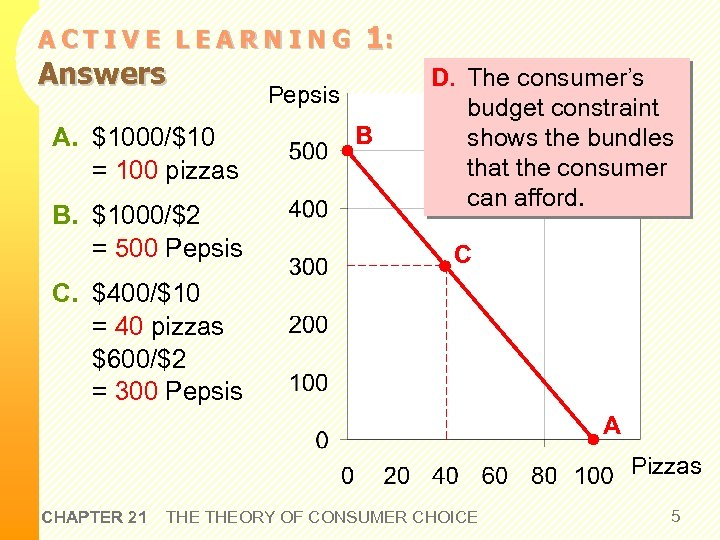ACTIVE LEARNING Answers A. \$1000/\$10 = 100 pizzas B. \$1000/\$2 = 500 Pepsis 1: Pepsis B D. The consumer’s budget constraint shows the bundles that the consumer can afford. C C. \$400/\$10 = 40 pizzas \$600/\$2 = 300 Pepsis A Pizzas CHAPTER 21 THEORY OF CONSUMER CHOICE 5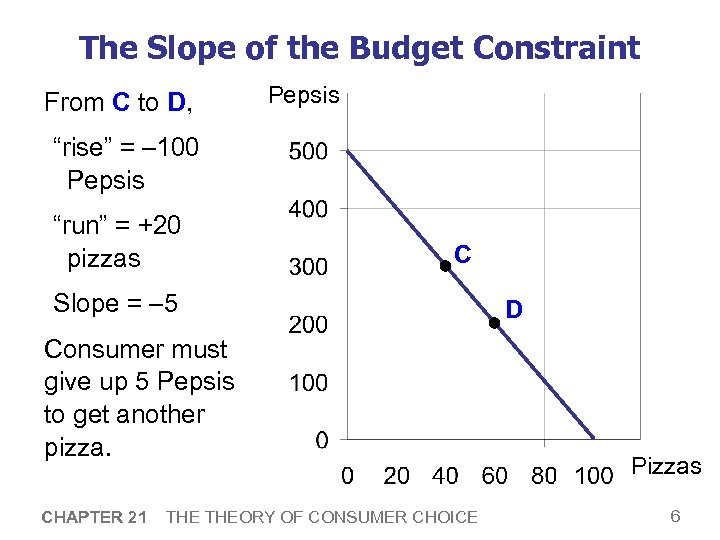The Slope of the Budget Constraint From C to D, Pepsis “rise” = – 100 Pepsis “run” = +20 pizzas C Slope = – 5 Consumer must give up 5 Pepsis to get another pizza. CHAPTER 21 THEORY OF CONSUMER CHOICE D Pizzas 6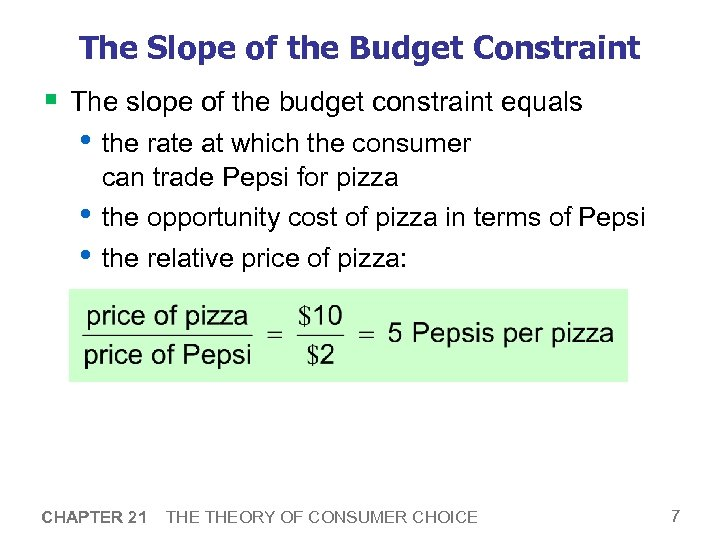The Slope of the Budget Constraint § The slope of the budget constraint equals • the rate at which the consumer can trade Pepsi for pizza • the opportunity cost of pizza in terms of Pepsi • the relative price of pizza: CHAPTER 21 THEORY OF CONSUMER CHOICE 7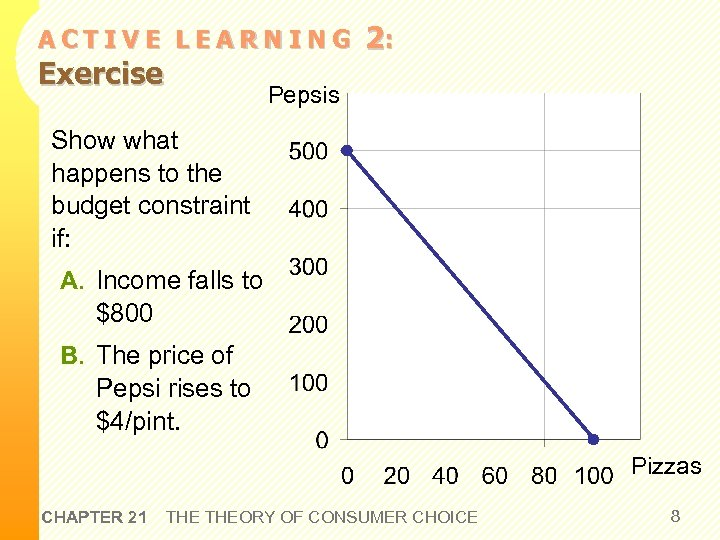ACTIVE LEARNING Exercise 2: Pepsis Show what happens to the budget constraint if: A. Income falls to \$800 B. The price of Pepsi rises to \$4/pint. Pizzas CHAPTER 21 THEORY OF CONSUMER CHOICE 8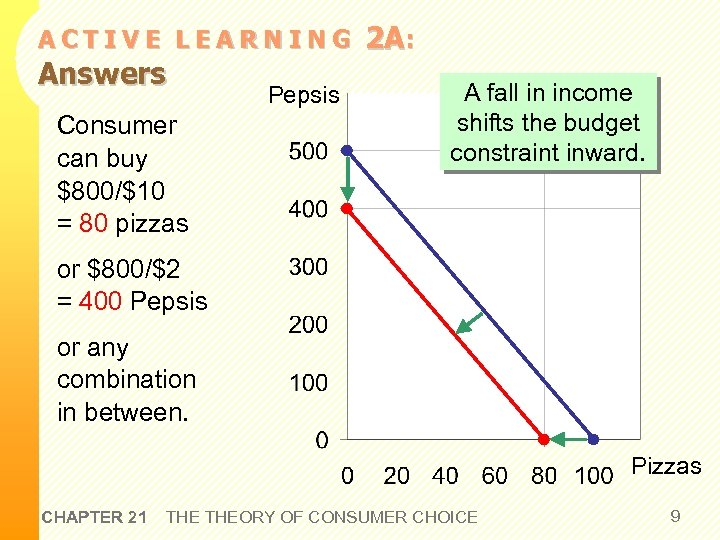ACTIVE LEARNING Answers Consumer can buy \$800/\$10 = 80 pizzas Pepsis 2 A: A fall in income shifts the budget constraint inward. or \$800/\$2 = 400 Pepsis or any combination in between. Pizzas CHAPTER 21 THEORY OF CONSUMER CHOICE 9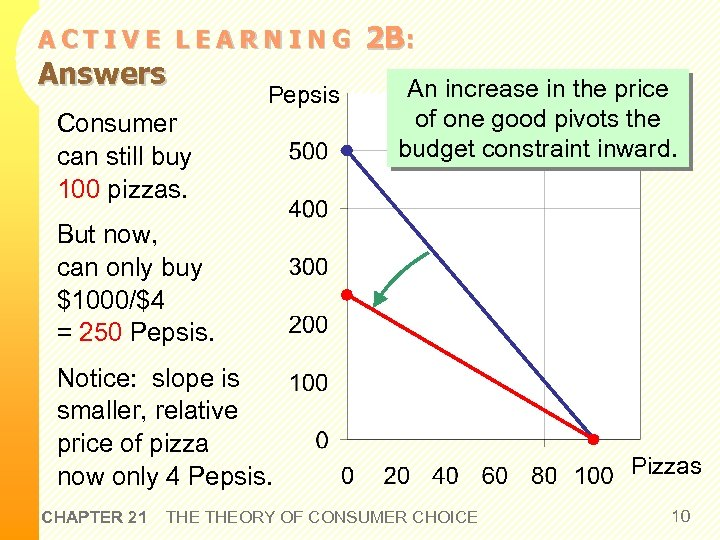ACTIVE LEARNING Answers Pepsis Consumer can still buy 100 pizzas. 2 B: An increase in the price of one good pivots the budget constraint inward. But now, can only buy \$1000/\$4 = 250 Pepsis. Notice: slope is smaller, relative price of pizza now only 4 Pepsis. CHAPTER 21 THEORY OF CONSUMER CHOICE Pizzas 10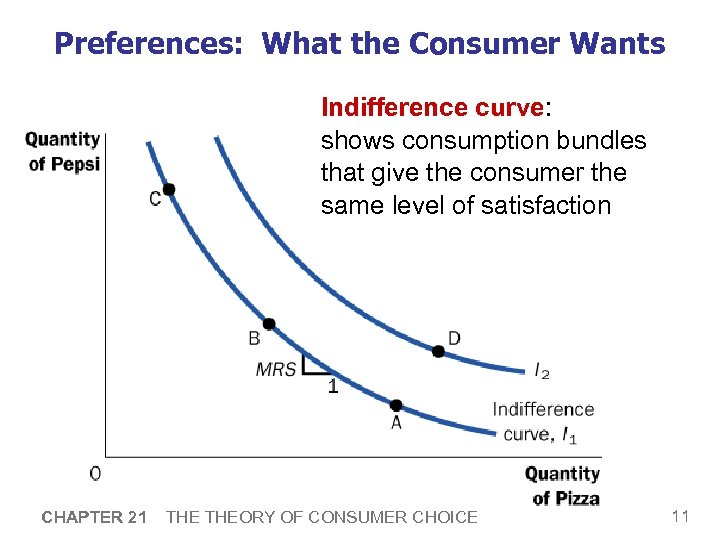Preferences: What the Consumer Wants Indifference curve: shows consumption bundles that give the consumer the same level of satisfaction CHAPTER 21 THEORY OF CONSUMER CHOICE 11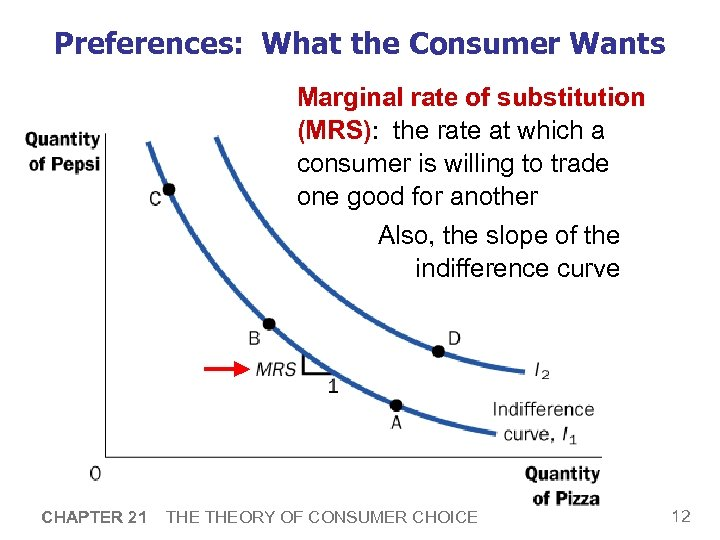Preferences: What the Consumer Wants Marginal rate of substitution (MRS): the rate at which a consumer is willing to trade one good for another Also, the slope of the indifference curve CHAPTER 21 THEORY OF CONSUMER CHOICE 12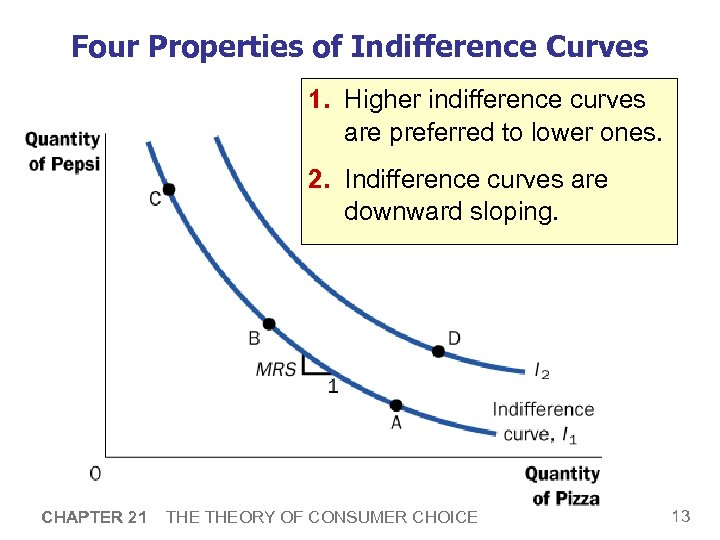Four Properties of Indifference Curves 1. Higher indifference curves are preferred to lower ones. 2. Indifference curves are downward sloping. CHAPTER 21 THEORY OF CONSUMER CHOICE 13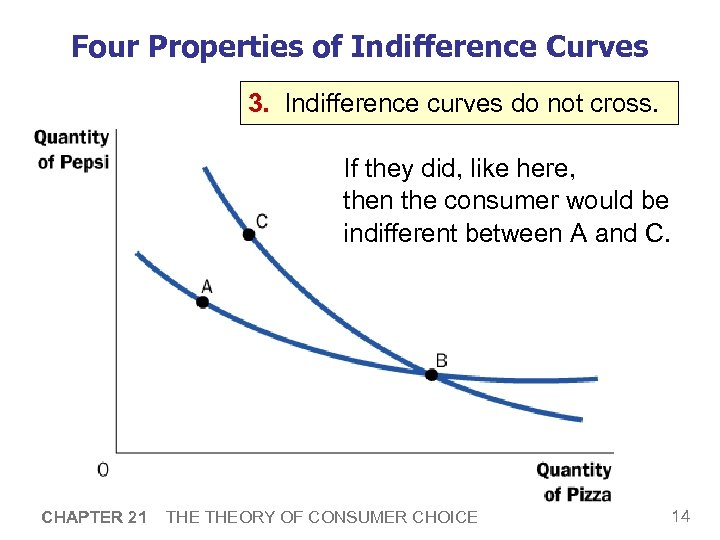Four Properties of Indifference Curves 3. Indifference curves do not cross. If they did, like here, then the consumer would be indifferent between A and C. CHAPTER 21 THEORY OF CONSUMER CHOICE 14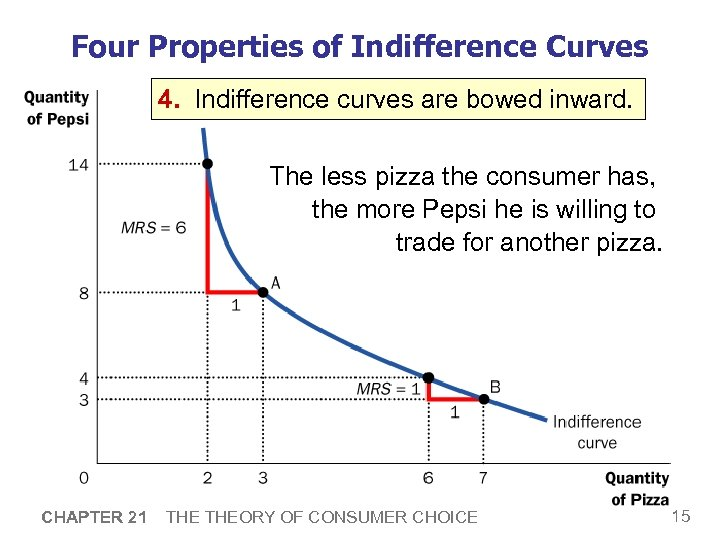Four Properties of Indifference Curves 4. Indifference curves are bowed inward. The less pizza the consumer has, the more Pepsi he is willing to trade for another pizza. CHAPTER 21 THEORY OF CONSUMER CHOICE 15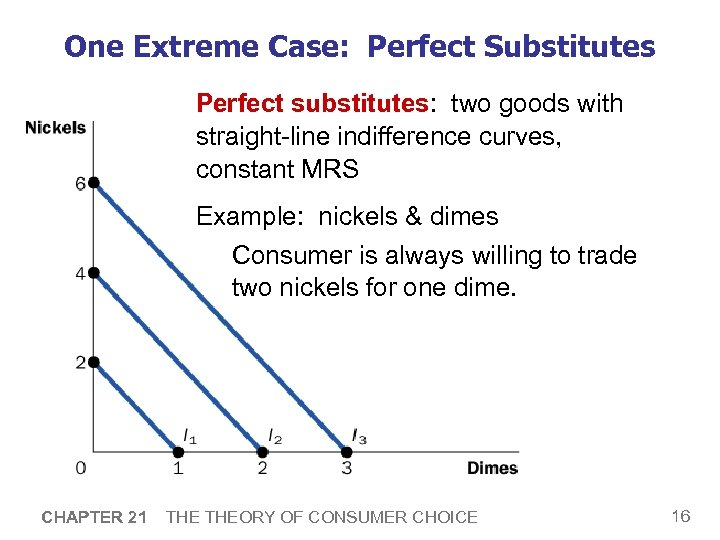One Extreme Case: Perfect Substitutes Perfect substitutes: two goods with straight-line indifference curves, constant MRS Example: nickels & dimes Consumer is always willing to trade two nickels for one dime. CHAPTER 21 THEORY OF CONSUMER CHOICE 16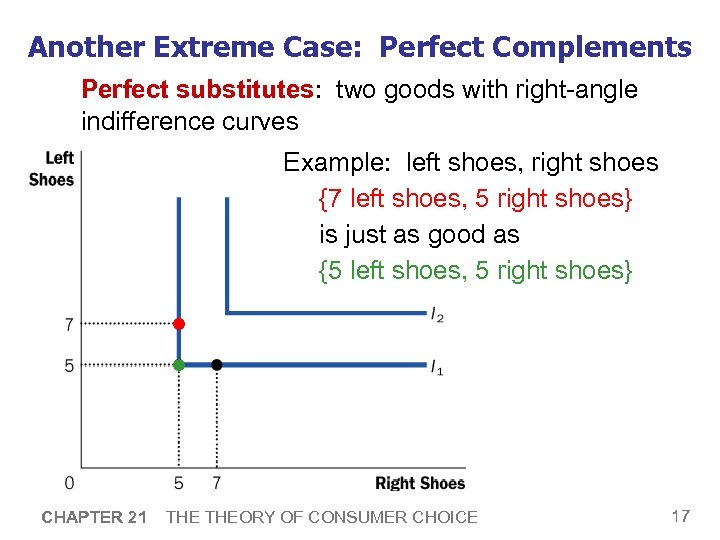Another Extreme Case: Perfect Complements Perfect substitutes: two goods with right-angle indifference curves Example: left shoes, right shoes {7 left shoes, 5 right shoes} is just as good as {5 left shoes, 5 right shoes} CHAPTER 21 THEORY OF CONSUMER CHOICE 17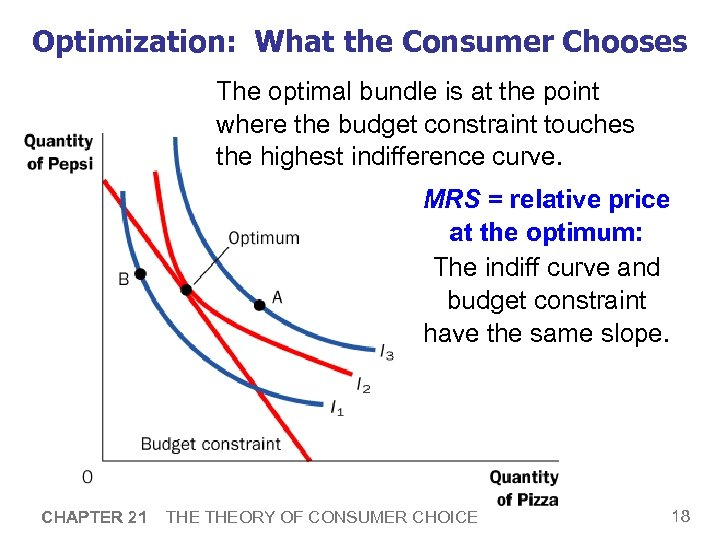Optimization: What the Consumer Chooses The optimal bundle is at the point where the budget constraint touches the highest indifference curve. MRS = relative price at the optimum: The indiff curve and budget constraint have the same slope. CHAPTER 21 THEORY OF CONSUMER CHOICE 18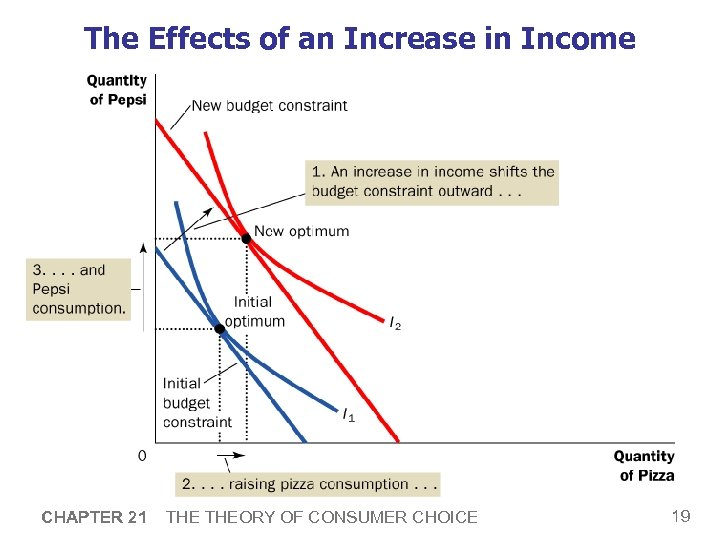The Effects of an Increase in Income CHAPTER 21 THEORY OF CONSUMER CHOICE 19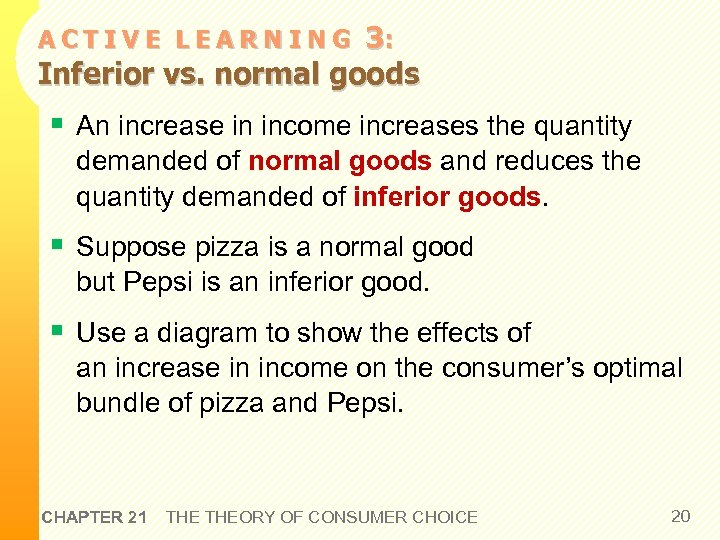3: Inferior vs. normal goods ACTIVE LEARNING § An increase in income increases the quantity demanded of normal goods and reduces the quantity demanded of inferior goods. § Suppose pizza is a normal good but Pepsi is an inferior good. § Use a diagram to show the effects of an increase in income on the consumer’s optimal bundle of pizza and Pepsi. CHAPTER 21 THEORY OF CONSUMER CHOICE 20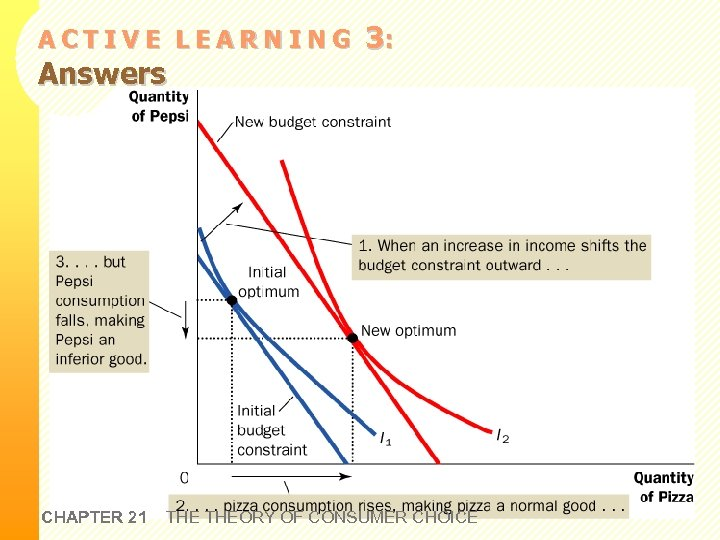ACTIVE LEARNING Answers CHAPTER 21 3: THEORY OF CONSUMER CHOICE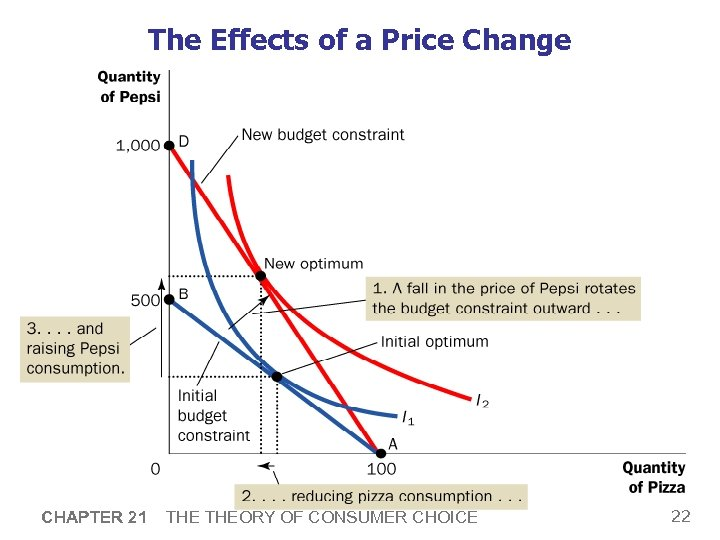The Effects of a Price Change CHAPTER 21 THEORY OF CONSUMER CHOICE 22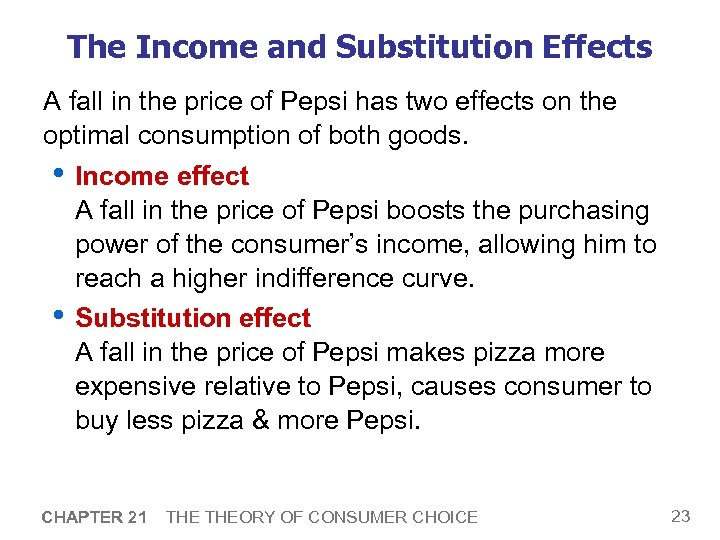The Income and Substitution Effects A fall in the price of Pepsi has two effects on the optimal consumption of both goods. • Income effect A fall in the price of Pepsi boosts the purchasing power of the consumer’s income, allowing him to reach a higher indifference curve. • Substitution effect A fall in the price of Pepsi makes pizza more expensive relative to Pepsi, causes consumer to buy less pizza & more Pepsi. CHAPTER 21 THEORY OF CONSUMER CHOICE 23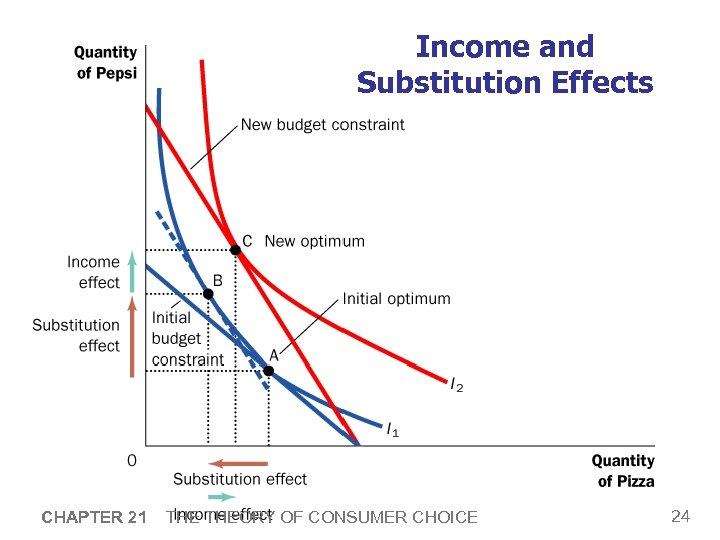Income and Substitution Effects CHAPTER 21 THEORY OF CONSUMER CHOICE 24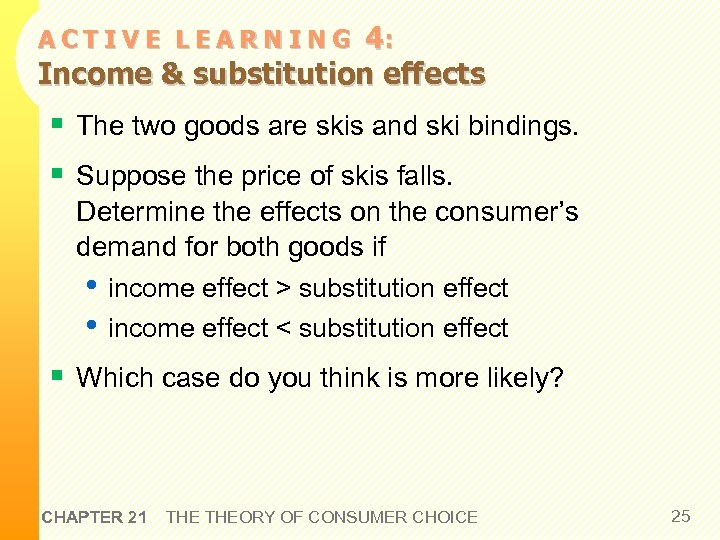4: Income & substitution effects ACTIVE LEARNING § The two goods are skis and ski bindings. § Suppose the price of skis falls. Determine the effects on the consumer’s demand for both goods if • income effect > substitution effect • income effect < substitution effect § Which case do you think is more likely? CHAPTER 21 THEORY OF CONSUMER CHOICE 25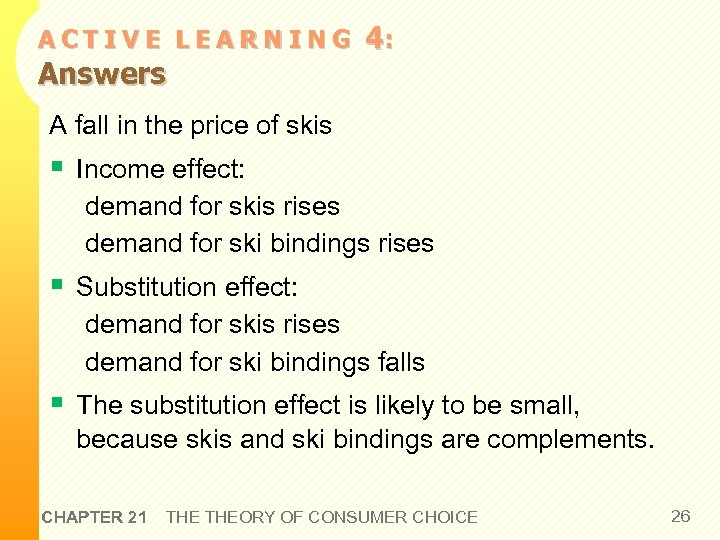ACTIVE LEARNING Answers 4: A fall in the price of skis § Income effect: demand for skis rises demand for ski bindings rises § Substitution effect: demand for skis rises demand for ski bindings falls § The substitution effect is likely to be small, because skis and ski bindings are complements. CHAPTER 21 THEORY OF CONSUMER CHOICE 26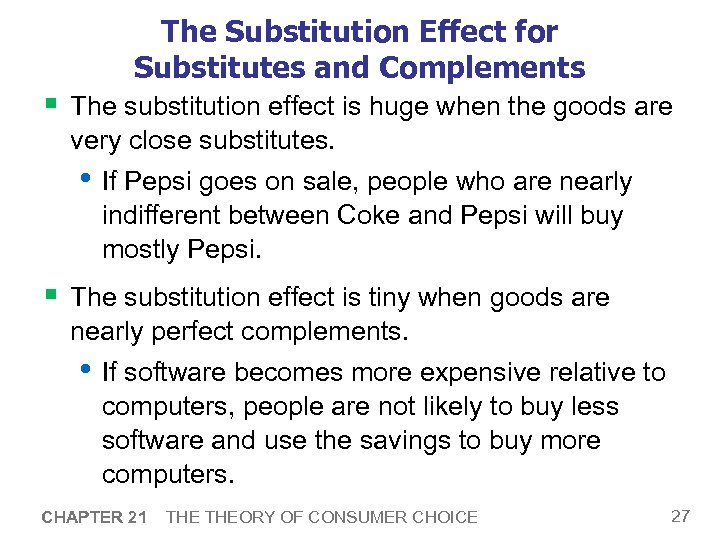The Substitution Effect for Substitutes and Complements § The substitution effect is huge when the goods are very close substitutes. • If Pepsi goes on sale, people who are nearly indifferent between Coke and Pepsi will buy mostly Pepsi. § The substitution effect is tiny when goods are nearly perfect complements. • If software becomes more expensive relative to computers, people are not likely to buy less software and use the savings to buy more computers. CHAPTER 21 THEORY OF CONSUMER CHOICE 27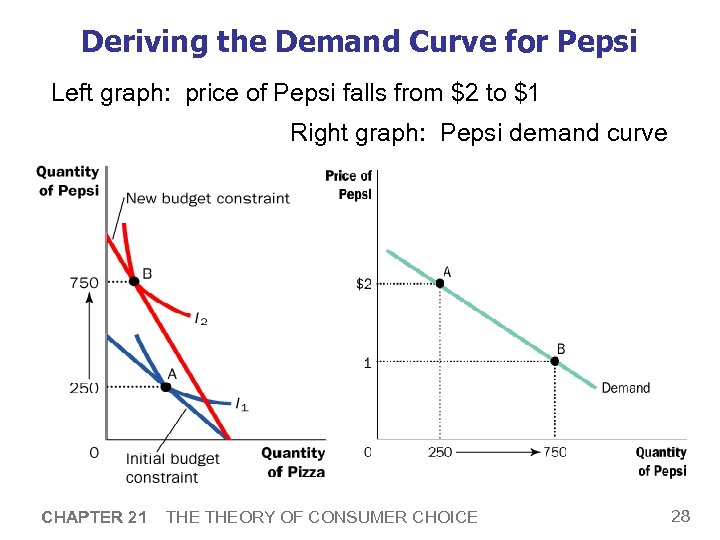Deriving the Demand Curve for Pepsi Left graph: price of Pepsi falls from \$2 to \$1 Right graph: Pepsi demand curve CHAPTER 21 THEORY OF CONSUMER CHOICE 28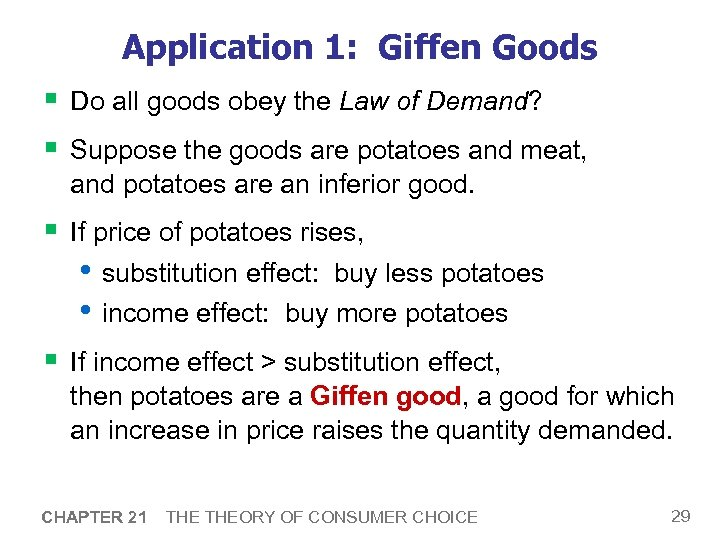Application 1: Giffen Goods § Do all goods obey the Law of Demand? § Suppose the goods are potatoes and meat, and potatoes are an inferior good. § If price of potatoes rises, • substitution effect: buy less potatoes • income effect: buy more potatoes § If income effect > substitution effect, then potatoes are a Giffen good, a good for which an increase in price raises the quantity demanded. CHAPTER 21 THEORY OF CONSUMER CHOICE 29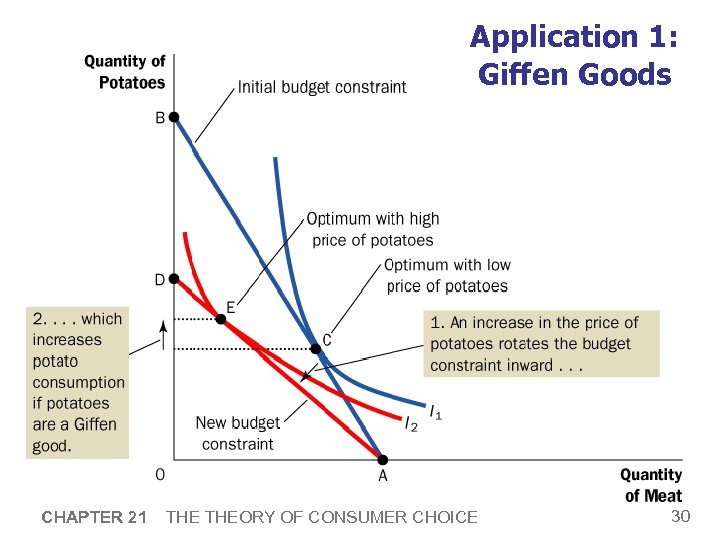Application 1: Giffen Goods CHAPTER 21 THEORY OF CONSUMER CHOICE 30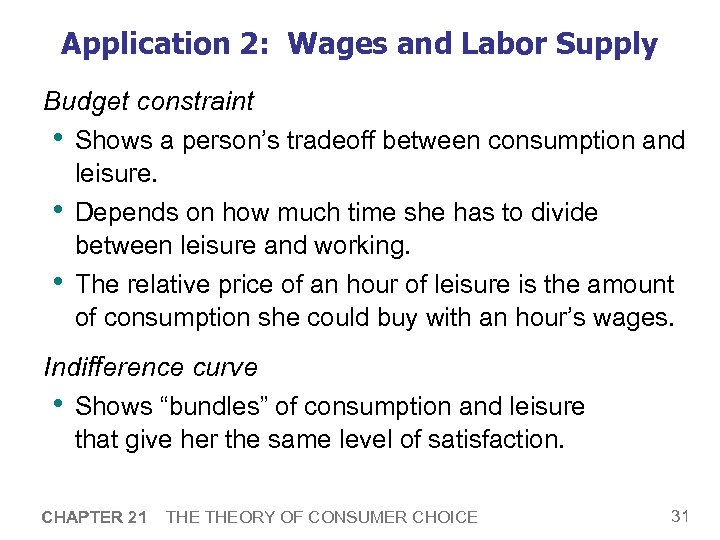Application 2: Wages and Labor Supply Budget constraint • Shows a person’s tradeoff between consumption and leisure. • Depends on how much time she has to divide between leisure and working. • The relative price of an hour of leisure is the amount of consumption she could buy with an hour’s wages. Indifference curve • Shows “bundles” of consumption and leisure that give her the same level of satisfaction. CHAPTER 21 THEORY OF CONSUMER CHOICE 31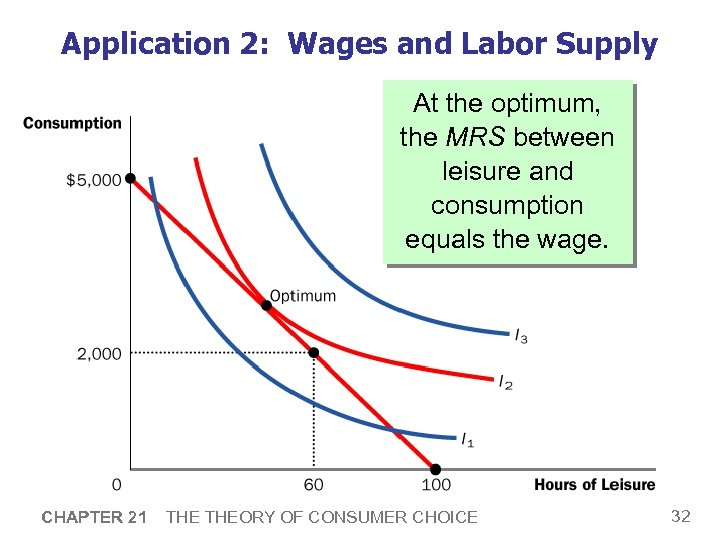Application 2: Wages and Labor Supply At the optimum, the MRS between leisure and consumption equals the wage. CHAPTER 21 THEORY OF CONSUMER CHOICE 32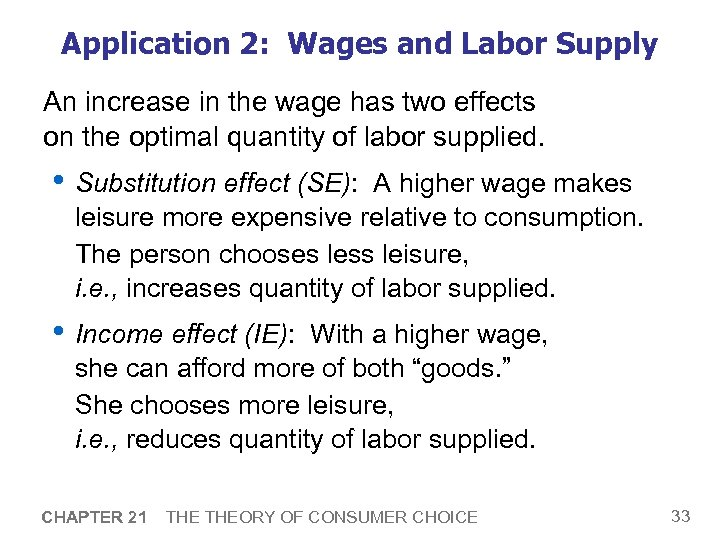Application 2: Wages and Labor Supply An increase in the wage has two effects on the optimal quantity of labor supplied. • Substitution effect (SE): A higher wage makes leisure more expensive relative to consumption. The person chooses less leisure, i. e. , increases quantity of labor supplied. • Income effect (IE): With a higher wage, she can afford more of both “goods. ” She chooses more leisure, i. e. , reduces quantity of labor supplied. CHAPTER 21 THEORY OF CONSUMER CHOICE 33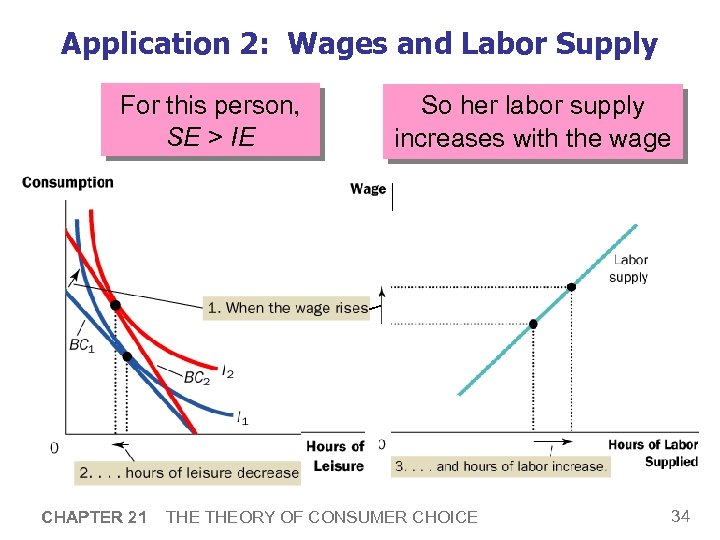Application 2: Wages and Labor Supply For this person, SE > IE CHAPTER 21 So her labor supply increases with the wage THEORY OF CONSUMER CHOICE 34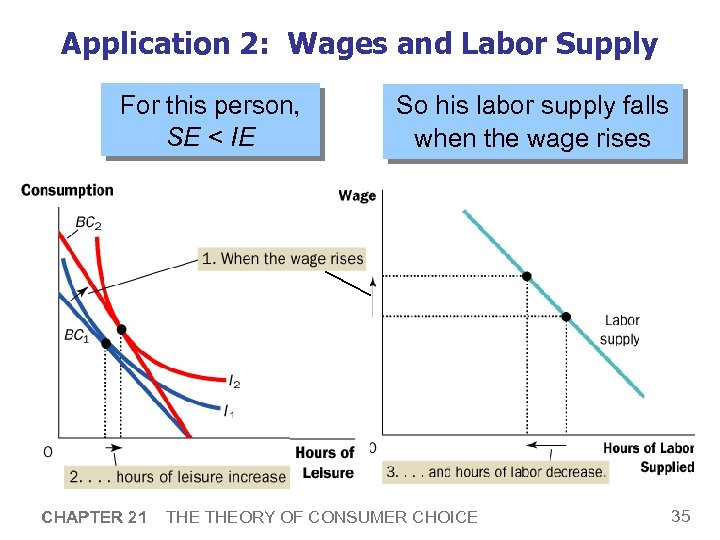Application 2: Wages and Labor Supply For this person, SE < IE CHAPTER 21 So his labor supply falls when the wage rises THEORY OF CONSUMER CHOICE 35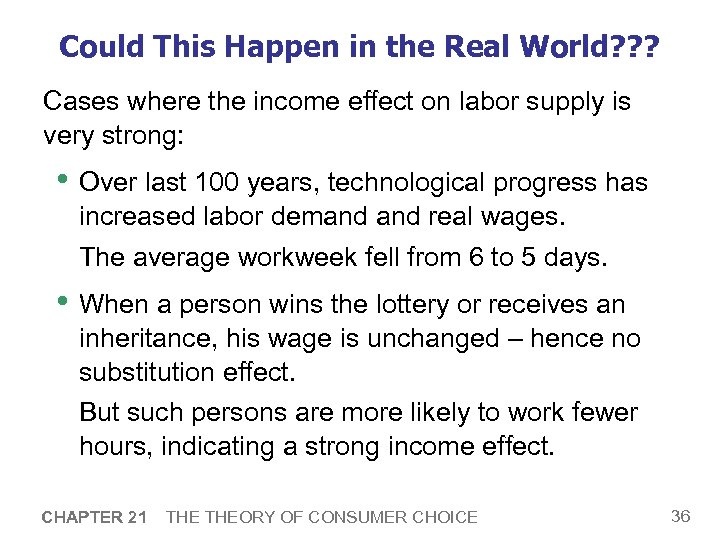Could This Happen in the Real World? ? ? Cases where the income effect on labor supply is very strong: • Over last 100 years, technological progress has increased labor demand real wages. The average workweek fell from 6 to 5 days. • When a person wins the lottery or receives an inheritance, his wage is unchanged – hence no substitution effect. But such persons are more likely to work fewer hours, indicating a strong income effect. CHAPTER 21 THEORY OF CONSUMER CHOICE 36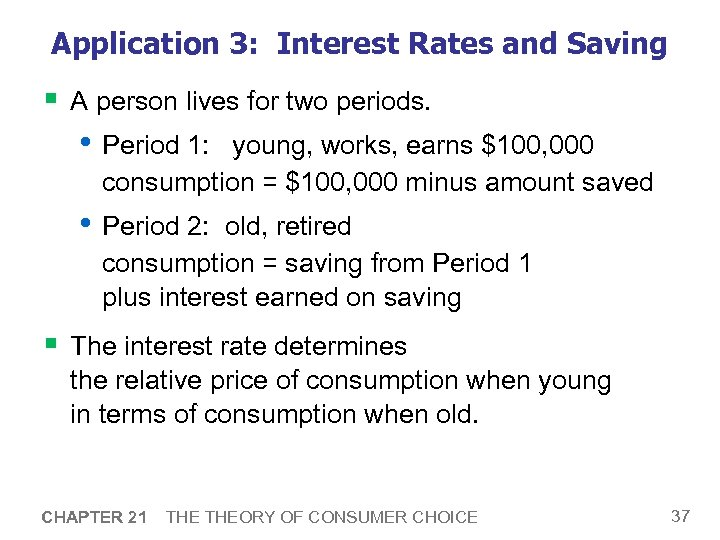Application 3: Interest Rates and Saving § A person lives for two periods. • Period 1: young, works, earns \$100, 000 consumption = \$100, 000 minus amount saved • Period 2: old, retired consumption = saving from Period 1 plus interest earned on saving § The interest rate determines the relative price of consumption when young in terms of consumption when old. CHAPTER 21 THEORY OF CONSUMER CHOICE 37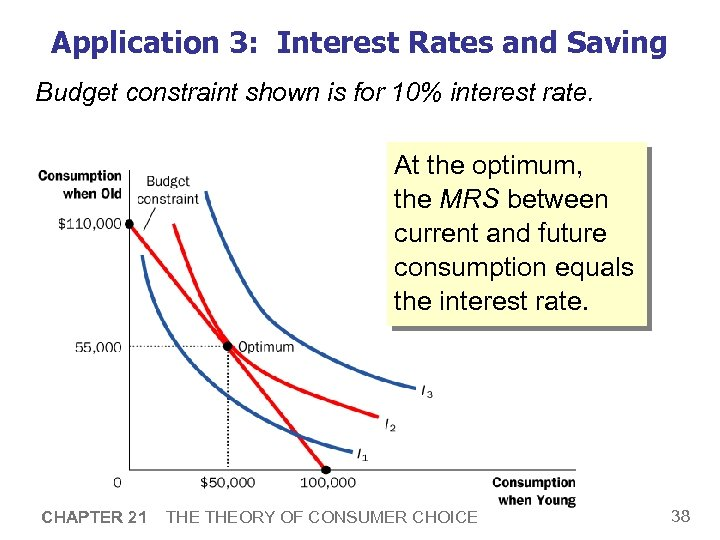Application 3: Interest Rates and Saving Budget constraint shown is for 10% interest rate. At the optimum, the MRS between current and future consumption equals the interest rate. CHAPTER 21 THEORY OF CONSUMER CHOICE 38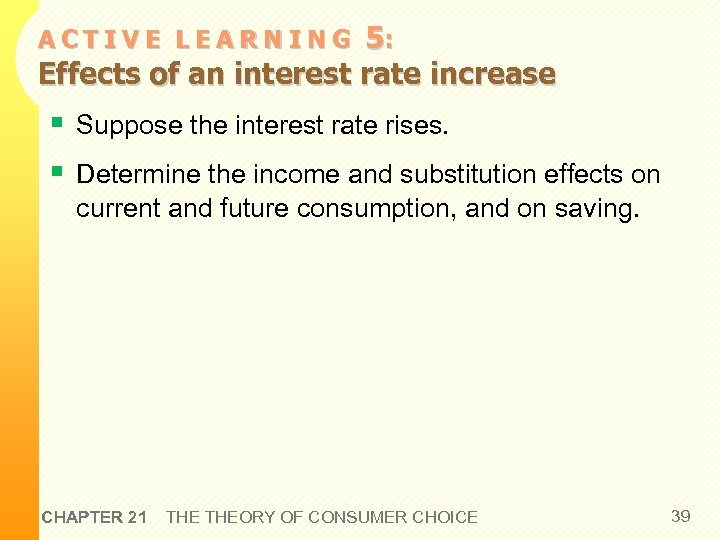5: Effects of an interest rate increase ACTIVE LEARNING § Suppose the interest rate rises. § Determine the income and substitution effects on current and future consumption, and on saving. CHAPTER 21 THEORY OF CONSUMER CHOICE 39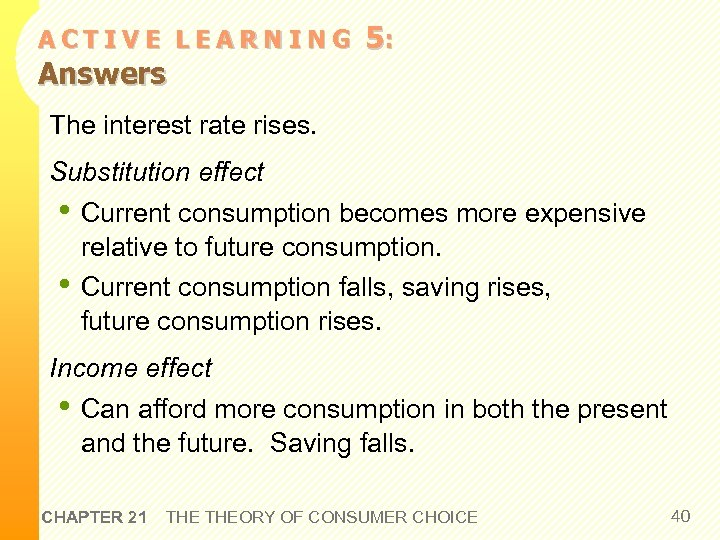ACTIVE LEARNING Answers 5: The interest rate rises. Substitution effect • Current consumption becomes more expensive • relative to future consumption. Current consumption falls, saving rises, future consumption rises. Income effect • Can afford more consumption in both the present and the future. Saving falls. CHAPTER 21 THEORY OF CONSUMER CHOICE 40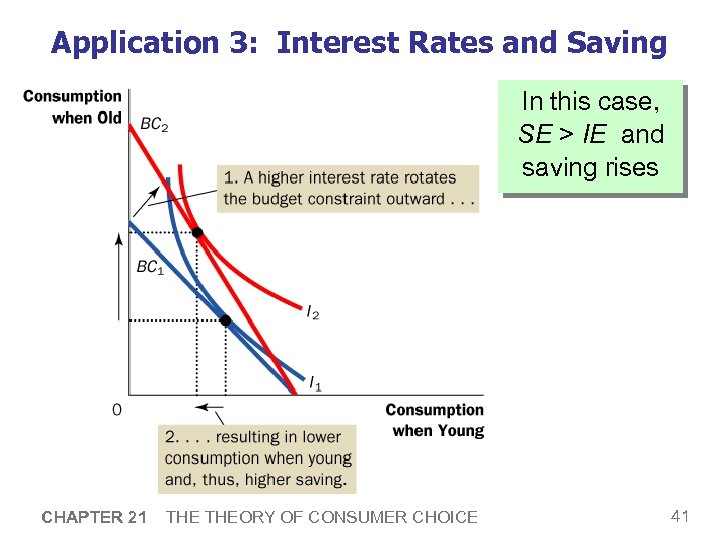Application 3: Interest Rates and Saving In this case, SE > IE and saving rises CHAPTER 21 THEORY OF CONSUMER CHOICE 41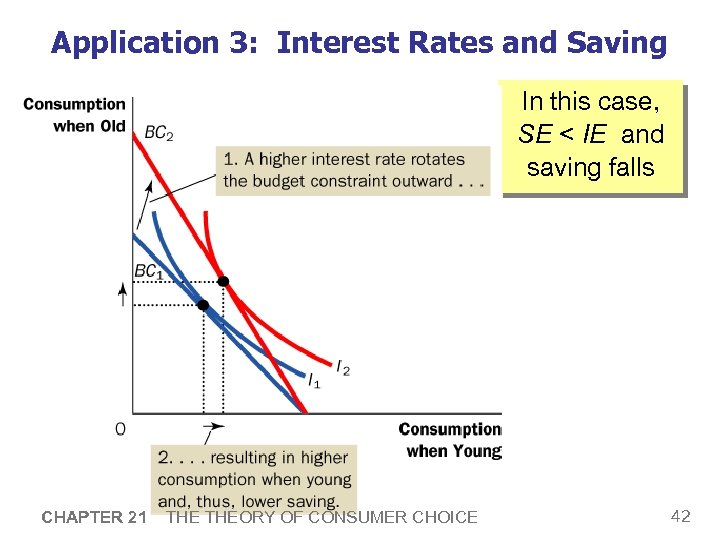Application 3: Interest Rates and Saving In this case, SE < IE and saving falls CHAPTER 21 THEORY OF CONSUMER CHOICE 42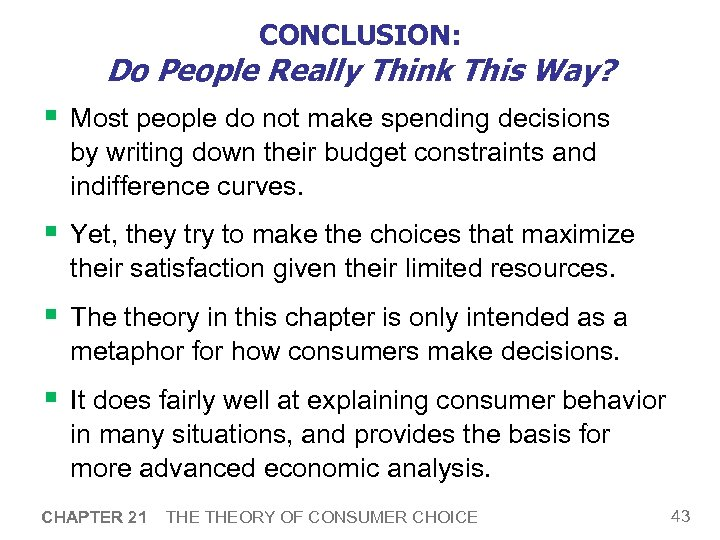CONCLUSION: Do People Really Think This Way? § Most people do not make spending decisions by writing down their budget constraints and indifference curves. § Yet, they try to make the choices that maximize their satisfaction given their limited resources. § The theory in this chapter is only intended as a metaphor for how consumers make decisions. § It does fairly well at explaining consumer behavior in many situations, and provides the basis for more advanced economic analysis. CHAPTER 21 THEORY OF CONSUMER CHOICE 43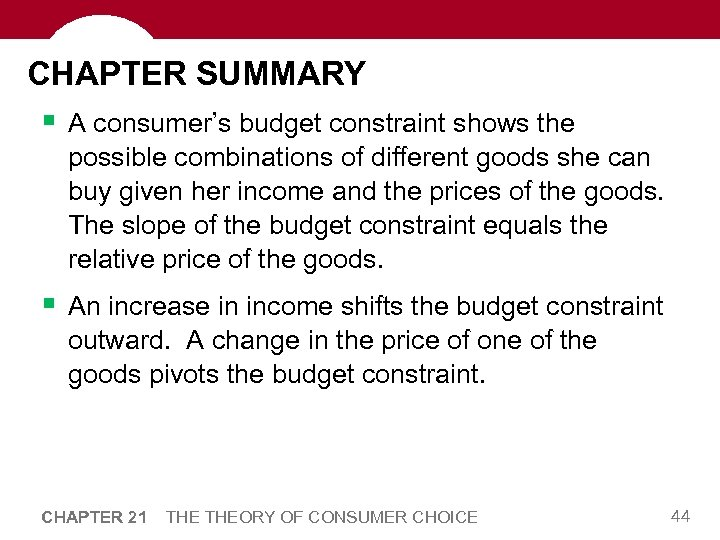CHAPTER SUMMARY § A consumer’s budget constraint shows the possible combinations of different goods she can buy given her income and the prices of the goods. The slope of the budget constraint equals the relative price of the goods. § An increase in income shifts the budget constraint outward. A change in the price of one of the goods pivots the budget constraint. CHAPTER 21 THEORY OF CONSUMER CHOICE 44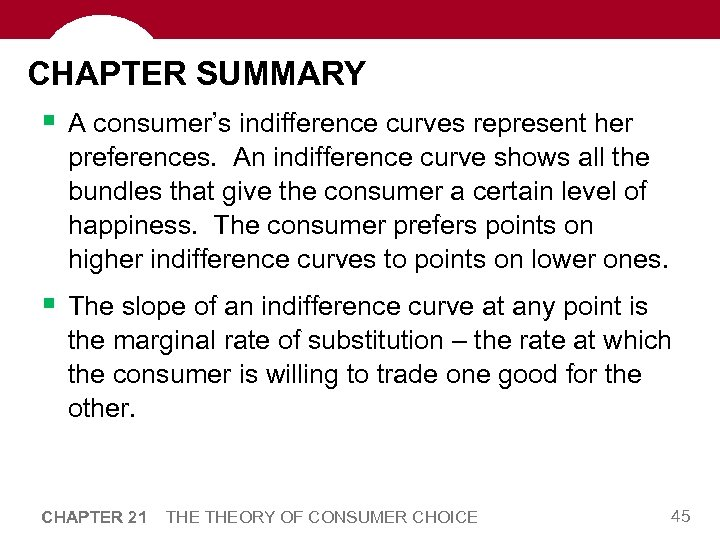CHAPTER SUMMARY § A consumer’s indifference curves represent her preferences. An indifference curve shows all the bundles that give the consumer a certain level of happiness. The consumer prefers points on higher indifference curves to points on lower ones. § The slope of an indifference curve at any point is the marginal rate of substitution – the rate at which the consumer is willing to trade one good for the other. CHAPTER 21 THEORY OF CONSUMER CHOICE 45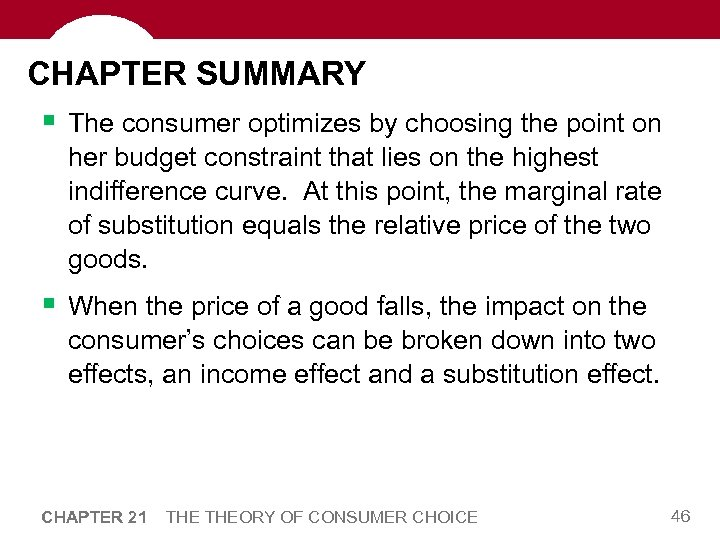CHAPTER SUMMARY § The consumer optimizes by choosing the point on her budget constraint that lies on the highest indifference curve. At this point, the marginal rate of substitution equals the relative price of the two goods. § When the price of a good falls, the impact on the consumer’s choices can be broken down into two effects, an income effect and a substitution effect. CHAPTER 21 THEORY OF CONSUMER CHOICE 46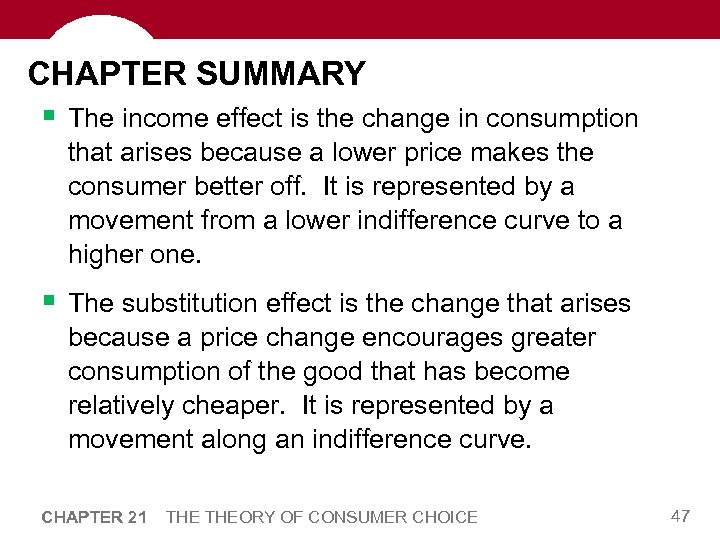CHAPTER SUMMARY § The income effect is the change in consumption that arises because a lower price makes the consumer better off. It is represented by a movement from a lower indifference curve to a higher one. § The substitution effect is the change that arises because a price change encourages greater consumption of the good that has become relatively cheaper. It is represented by a movement along an indifference curve. CHAPTER 21 THEORY OF CONSUMER CHOICE 47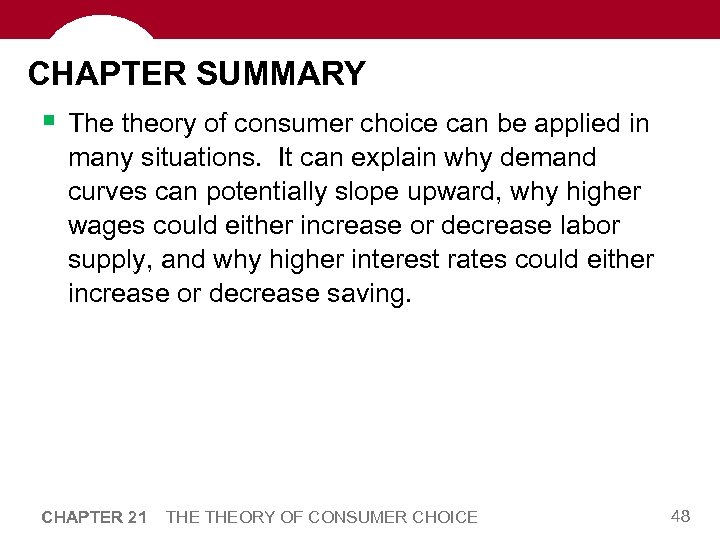CHAPTER SUMMARY § The theory of consumer choice can be applied in many situations. It can explain why demand curves can potentially slope upward, why higher wages could either increase or decrease labor supply, and why higher interest rates could either increase or decrease saving. CHAPTER 21 THEORY OF CONSUMER CHOICE 48